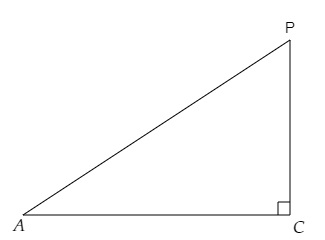# If $\angle A$ and $\angle P$ are acute angles such that $\tan A=\tan P$, then show that $\angle A=\angle P$.

Given:

$\angle A$ and $\angle P$ are acute angles such that $\tan A=\tan P$.

To do:

We have to show that $\angle A=\angle P$.

Solution:

Let, in a triangle $APC$ right angled at $C$, $tan\ A = tan\ P$.We know that,

In a right-angled triangle $APC$ with right angle at $C$,

By trigonometric ratios definitions,

$tan\ A=\frac{Opposite}{Adjacent}=\frac{PC}{AC}$

$tan\ P=\frac{Opposite}{Adjacent}=\frac{AC}{PC}$

This implies,

$\tan A=\tan P$

$\Rightarrow \frac{PC}{AC}=\frac{AC}{PC}$

$\Rightarrow PC \times PC=AC \times AC$

$\Rightarrow PC^2 =AC^2$

$\Rightarrow PC = AC$

We know that,

Angles opposite to equal sides are equal in a triangle.

Therefore,

$\angle A=\angle P$

Hence proved.